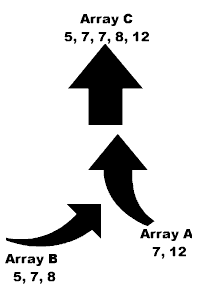Merge SortThe merge sort combines two sorted arrays into one larger sorted array.   As the diagram at the left shows, Array A and Array B merge to form Array C.  Arrays to be merged MUST be SORTED FIRST!!  Be sure to declare Array C  in main( ) and establish its size. Example: Ascending Order Array A: {7. 12} Array B: {5,  7, 8} Array C: {5, 7, 7, 8, 12} after merge

Here is how it works:  The first element of array A is compared with the first element of array B.  If the first element of array A is smaller than the first element of array B, the element from array A is moved to the new array C.  The subscript of array A is now increased since the first element is now set and we move on.

If the element from array B should be smaller, it is moved to the new array C.  The subscript of array B is increased.  This process of comparing the elements in the two arrays continues until either array A or array B is empty.  When one array is empty, any elements remaining in the other (non-empty) array are "pushed" into the end of array C and the merge is complete.

//Function to merge two pre-sorted arrays
void MergeSort(apvector <int> &arrayA, apvector <int> &arrayB, apvector <int> &arrayC)
{
int indexA = 0;     // initialize variables for the subscripts
int indexB = 0;
int indexC = 0;

while((indexA < arrayA.length( )) && (indexB < arrayB.length( ))
{
if (arrayA[indexA] < arrayB[indexB])
{
arrayC[indexC] = arrayA[indexA];
indexA++;    //increase the subscript
}
else
{
arrayC[indexC] = arrayB[indexB];
indexB++;      //increase the subscript
}
indexC++;      //move to the next position in the new array
}
// Move remaining elements to end of new array when one merging array is empty
while (indexA < arrayA.length( ))
{
arrayC[indexC] = arrayA[indexA];
indexA++;
indexC++;
}
while (indexB < arrayB.length( ))
{
arrayC[indexC] = arrayB[indexB];
indexB++;
indexC++;
}
return;
}